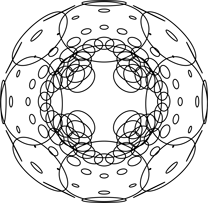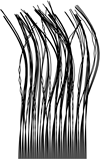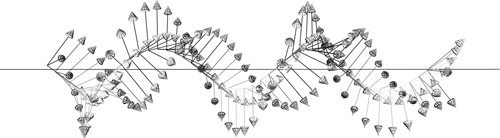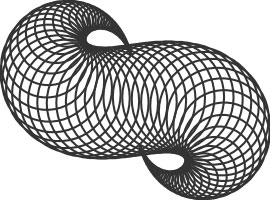# Versor (libvsr)

## A (fast) Generic C++ library for Geometric Algebras, including Euclidean, Projective, Conformal, Spacetime (etc).

### Currently tested on Linux and Mac OS X

Homepage (versor.mat.ucsb.edu)

Documentation

Combinatorics Cheat Sheet

Bibtex (for referencing this work in your paper)

Versor is a C++ Library for Geometric Algebra, sometimes called Clifford Algebra, a system for encoding geometric concepts numerically.

The headers generate optimized code at compile-time through template metaprogramming. The core of the library is under 150kb, and supports arbitrary dimensions and metrics (limited by your compiler…).

The library can be used as a math-only, or as an application with built-in graphics. Both OpenGL and OpeGLES draw routines are supported.

gmail: wolftype

## Recent Changes

Some major revisions have been enacted that change the API.

• namespaces are used to distinguish between algebras:
• vsr::nga:: is the namespace for generic n-dimensional functions
• vsr::cga:: is the namespace for 3D Conformal Geometric Algebra
• For example vsr::nga::Round:: calls the generic implementation of n-D round elements whereas vsr::cga::Round:: calls the 3D CGA specification
• all static function structs (Round::, Flat::, Tangent::) are written in full (as opposed to Ro::, Fl:: and Ta::). We note these are Capitalized, since earlier versions of the devel branch used lowercase namespaces.
• Construct:: is now used to construct useful objects within a particular namespace
• example: Construct::point(0,1,0) constructs a point at coordinate (0,1,0)
• header folders /detail /space /form /draw and /util organize the various files

## PUBS:

My disseration, Articulating Space, introduces geometric algebra and explores applications to parametric design. My Master’s Thesis before it, serves as an introduction as well.

At C++ Now in Aspen I gave a Presentation on Metaprogramming Implementation Details

As long as algebra and geometry have been separated, their progress have been slow and their uses limited; but when these two sciences have been united, they have lent each mutual forces, and have marched together towards perfection.
-Joseph Louis Lagrange

No attention should be paid to the fact that algebra and geometry are different in appearance.
-Omar Khayyám

L’algèbre n’est qu’une géométrie écrite; la géométrie n’est qu’une algèbre figurée.
-Sophie Germain

If you want to see, learn how to act
-Heinz von Foerster


## OTHER NICE SOFTWARE:

• Cinderella Standalone GA interface
• GAViewer Standalone GA interface
• Gaigen An Implementation Generator
• CluCalc/CluViz Standalone and Library
• Gaalop A precompiler to optimize CluScripts
• Gaalet An expression template library whose backend is somewhat similar to Versor’s
• versor.js A javascript port of this library

## COMPILATION

For this version you need C++11 support, which is now common (gcc 4.7 or higher or clang 3.2 or higher) and for graphics support you’ll want glew.
See the Troubleshooting section below for instructions on installing glew.

git clone https://github.com/wolftype/versor.git


You’ll need to initialize the submodules to build any graphics examples:

cd versor
git submodule init
git submodule update


To build library and all programs in /examples:

./build.sh


To build library only without examples:

./build.sh --lib


To build library without graphics:

./build.sh --math


To compile and run programs in scratch/projects:

./run.sh scratch/projects/<folder>/<filename>


Note that not all projects will compile as I am in the process of updating them in this development branch.

Adding “configure” to the end of the above ./run.sh command first re-runs cmake in order to add <filename> to its list of targets. For instance, to build and run programs you have written (e.g. in some folder called scratch/projects/user/), you can first write:

./run.sh scratch/projects/user/<filename> --configure


This need only happen once when you create a new file. Thereafter you can run your file without adding “configure” to the end

## 1. A math library:

#include "vsr.h"

using Vec = vsr::euclidean_vec<3,float>;        //<-- A 3 dimensional euclidean vector defined over floats
using Biv = vsr::euclidean_bivector<3,float>;   //<-- A 3 dimensional bivector or "directed area element"

int main(){

auto v = Vec(1,2,3);                    //<-- A 3D vector at coordinates 1,2,3;

v.rotate( Biv::xy * .25 ).print();      //<-- Rotate the vector in the xy plane and print result

return 0;
};


## 2. A stand-alone application (with window and gui)

While fully enabling arbitrary metric spaces, Versor has a lot of built-in functionality for specifically working with Conformal Geometric Algebra of 3D space, which is THE way to model all Euclidean transformations:

Point p = Round::point(0,0,0);

auto tp = p.translate(x, y, z);

auto rp = p.rotate( Biv::xy * theta)


## TROUBLESHOOTING

• You may need to install Glew on newer macs. Best way to do this is with brew:

brew install glew

• You’ll need C++11 support on your compiler (See makefile notes below). For C++11 you’ll want clang 3.2 (mac) or above or gcc 4.7 or above (linux).

• Alternatively an earlier version of Versor is available at github.com/wolftype/versor_1.0.git This older version runs just as fast, but is strictly 3D CGA (i.e. R4,1 metric) since I generated headers ahead of time.

## INTRODUCTION

Versor provides operations and draw routines for Euclidean and Conformal Geometric Algebras, a relatively new spatial computing model used by physicists, engineers, and artists. Versor is designed to make graphical experimentation of geometric algebra within a C++ environment easier. You can use this library to draw geometrical things, explore spherical and hyperbolic spaces, transformations, design robots, etc. I am using it for my PhD on bio-inspired engineering.

I first developed Versor while reading “Geometric Algebra for Computer Science” by Leo Dorst, Daniel Fontijne, and Stephen Mann. It’s a fantastic book and if you’re reading this you should also consider reading that.

Built to aid in my modelling of organic forms, the initial development was partially funded by the Olivia Long Converse Fellowship for Botanic research, courtesy of the Graduate Division at the University of California in Santa Barbara. It has also received support from the Robert W. Deutsch Foundation.

One quick word: clifford algebras and the spatial relationships they embody can often feel abstract and daunting. But it’s a twisty, boosty ride, full of weird discoveries. You’re bound to make some, so have fun!

#### BACKGROUND

The homogenous 5D CGA model used here was initially proposed by David Hestenes, Hongbo Li, and Alan Rockwood in 2001, and given full form and weight through the excellent and careful work of Leo Dorst, Joan and Anthony Lasenby, and Eduardo Bayro-Corrochano, and others. These researchers’ writings have helped me quite a bit. CGA is particular breed of Clifford Algebras (also known as Geometric Algebras), which operate upon combinatoric hypercomplex vector spaces that emerged from William Clifford’s attempt to fuse Hamilton’s quaternions with Grassmans’ extension algebras. Thus transformations were married with a system of abstraction. For more information, take a look at the links to the sites at the bottom of this page. For instance, for practical applications in robotics and “Geometric Cybernetics”, see Eduardo Bayro-Corrochano’s work. For some very helpful algorithms in rigid body dynamics and gravitational physics see the variety of publications by Joan and Anthony Lasenby. To get at the beginning of it all, read David Hestenes’ New Foundations for Classical Mechanics.

#### SPEED

Typical matrix operation libraries have templated inlined functions for Vector and Matrix multiplication. Versor is similar, but on steroids, where vectors and sparse matrices of various sizes are all just called multivectors and represent geometric elements beyond just xyz directions and transformation matrices. Circles, lines, spheres, planes, points are all algebraic elements, as are operators that spin, twist, dilate, and bend those variables. Both these elements and operators are multivectors which multiply together in many many many different ways.

#### What’s new?

Versor compiles much faster than before, and without any silly predetermined list of allowable operations or types. Most notably, arbitrary metrics are now possible. For example, the xRoots.cpp example calculates all the Euclidean 4D reflections of a couple of point groups (F4 and D4, namely). So you can hypercube and polychoron away (8D cubes no problem!). Number of dimensions allowed are somewhat limited by your compiler infrastructure — let me know if you have a need that is not being met!

As for CGA, all the Pnt, Vec, Dll notation remains as before, but i’ve started adding utility functions since it helps people out.

auto pa = Round::point( 1,0,0 );
auto pb = Round::point( 0,1,0 );
auto pc = Round::point(-1,0,0 );
auto circle = pa ^ pb ^ pc;

Draw(c);


#### How does it work?

If you like functional template metaprogramming, take a look at the code and please let me know what you think. If you don’t, then I wouldn’t …
But if you have ideas or questions please do not hesitate to contact me.

## WHAT THE POINT IS

GA combines many other maths (matrix, tensor, vector, and lie algebras). It is holistic. CGA uses a particular mapping (a conformal one) of 3D Euclidean space to a 4D sphere. Operations on that hypersphere are then projected back down to 3D. That how it works in a nutshell.

A fuller treatment of this question (er, the question of why we do this) can be found in my Master’s thesis on the subject. But basically, Geometic Algebra offers a particular richness of spatial expression. Imagine needing glasses and not knowing you needed glasses. Then, when you do get glasses, the world changes unexpectedly. GA is like glasses for the inside of your brain. Conformal Geometric Algebra, especially the 5D variety enlisted here, are like x-ray glasses. One point of clarification that occurs are disambiguations of previously collapsed concepts.

For instance, the main disambiguation, is that between a Point in space and a Vector in space.
A Point has no magnitude, but a Vector does. A Point has no direction, but a Vector does. Points are null Vectors. We can make them by writing

Vec( 1,0,0 ).null();

• Points are null Vectors
• Points square to 0
• The dot (inner) product of two Points returns their squared distance
• The wedge (outer) product of two Points returns a Point Pair

More on that last point later … there are various binary operators defined (mainly three). We can introduce one right now, which is the dot or inner product. In mathematics, the inner product of two points pa and pb is written $$p{a} \rfloor p{b}$$. In Versor we use the <= operator:

Point pa = Vec(1,0,0).null();
Point pb = Vec(-1,0,0).null();
Scalar squaredDist = ( pa <= pb ) * -2;


which in this case would return a Scalar value of 4. The -2 is there since the inner product really returns half the negative squared distance. We can extract the Scalar into a c++ double like so:

double squaredDist = ( pa <= pb ) * -2;


Points thought of as Spheres (really, Dual Spheres, more on Duality later): they are Spheres of zero radius. As such they are a type of Round element. We can also build points this way:

Round::null( 1,0,0 );


or you can pass in another element

Round::null( Vec(1,0,0) );


or use the built-in method

Point pa = Vec(1,0,0).null();


Points can also be made with the macro PT

Point pa = PT(1,0,0);


which is just “syntactic sugar” for Vec(1,0,0).null()

Speaking of Spheres, we can also make spheres with a radius this way:

DualSphere dls = Round::dls( Vec( 1,0,0 ).null(), 1 );


or

DualSphere dls = Round::dls( Vec( 1,0,0 ), 1 );


or, specifying the radius first and then the coordinate:

DualSphere dls = Round::dls( 1 /* <--radius */ , 1,0,0 )


all of which give a dual sphere of radius 1 at coordinate 1,0,0;

## BASICS

Versor is named after the one of the basic category of elements of geometric algebra.
A versor is a type of multivector which can be used to compose geometric transformations, namely reflections, translations, rotations, twists, dilations, and transversions (special conformal transformations).

More on all of those transformations later.

In Versor, a Vector (or Vec) is a typical Euclidean 3D element. It can be built in the normal way:

Vec v(1,2,3);


Some built-in Vectors exist:

Vec::x x; //<-- X Direction Unit Vector Vec(1,0,0)
Vec::y y; //<-- Y Direction Unit Vector Vec(0,1,0)
Vec::z z; //<-- Z Direction Unit Vector Vec(0,0,1)


A Vector can be spun around using a Rotor, which is exactly like a quaternion. However, whereas quaternions are often built by specifying an axis and an angle, rotors are built by specifying the plane of rotation. Eventually this will make much more sense to you: in general planes are what we will be using to transform things. For instance, a reflection is a reflection in a plane. As we will see, planes can become hyperplanes which will allow for more extraordinary transformations.

The first completely new element to introduce is the Bivector, which is the plane we will use to generate our Rotor. Bivectors represent directed areas and are dual to the cross product: the cross product of two vectors in typical vector algebra returns a vector normal to the plane they define. So it is not completely new, but just sort of new.

Bivectors are also just three elements long, and are built the same way Vectors are.

Biv b(1,2,3);


Some built-in Bivectors exist:

Biv::xy xy; //<-- XY Counterclockwise Unit Area Biv(1,0,0)
Biv::xz xy; //<-- XZ Counterclockwise Unit Area Biv(0,1,0)
Biv::yz xy; //<-- YZ Counterclockwise Unit Area Biv(0,0,1)


While it is perfectly valid to write Vector, Bivector and Rotor, you’ll notice I’ve truncated them to their three letter nicknames, Vec and Rot.
That’s up to you: Both long-name and nick-name versions are valid in libvsr (they are typedef’ed to each other).

Biv b = Biv::xy;
double theta = PIOVERTWO;
Vec v1 = Vec::x.rot( b * theta )


You can also generate rotors using Gen::rot( <some bivector> ) In fact, all transformations can be generated this way, and then later applied to arbitrary elements. For instance, Motors can be generated which translate and rotate an element at the same time. This is also called a twist.

Motor m = Gen::mot(<some dual line>);   //<-- Makes A Twisting Motor around Some Dual Line
Point p = Vec(0,0,0).null().sp(m);      //<-- Applies above motor to a Point


You’ll notice there are dual versions of elements: as in a DualLine (or Dll for short). That’s because in the real world of abstract geometry, there are usually two ways of defining an element. For instance, we can build a direct Line on the Y-axis by wedging two points together, along with infinity:

Line lin = Vec(0,0,0).null() ^ Vec(0,1,0).null() ^ Inf(1);


Or we can define a line by the bivector plane that it is normal to, and a support vector that determines how far away the line is from the origin. To convert the above line into its dual representation, we just call the dual() method:

Dll dll = lin.dual();


For those who are interested, this dual representation is isomorphic to the Plücker coordinates, which are used in screw theory to twist things around. Here, too, we can use dual lines to generate transformations which twist things around them.

## Gui

The examples/*.cpp files include bindings to the GLV framework for windowing and user interface controls. This provides the minumum necessary glue to get started quickly building your own GA based graphics applications.

The interface has a built in gui, mouse info, and keyboard info stored.

//... a member of your App
Circle circle;
//...in App::setup()
objectController.attach(&circle);


Putting the above code inside your application will enable you to click and modify geometric elements by hitting the “T”, “R” and “S” keys (for translate, rotate, and scale) Hit any other key to deselect all elements.

[BUILT-IN INTERFACE]

| | | Key | Response
——————————————— | —————————————————————
~ | Toggle full screen.
SHIFT + Mouse or Arrow Keys | Translate the camera in x and z directions.
CTRL+ Mouse or Arrow Keys | Rotate the camera
ALT +Arrow Keys | Rotate the model view around.
T | Translate an Element
R | Rotate an Element
S | Scale an Element Tab | Switch from navigation mode (default) to object manipulation mode Any other key | Release all Elements

## OPERATORS

The elements of the algebra are geometric entities (circles, planes, spheres, etc) and operators (rotations, translations, twists, etc) which act on the elements of the algebra. All are known as multivectors since they are more than just your typical vectors.

Multivector elements are most often combined using three overloaded binary operators:

The Geometric Product of elements A and B:

A * B


multiplies two multivector elements together. This is most useful when multiplying one by the inverse of another (see ! operator, below).

The Outer Product of elements A and B:

A ^ B


“wedges” two multivectors together. Its from Grassman’s algebra of extensions, and can be thought of as a way of creating higher dimensions from smaller ones. For instance, wedging two Vectors (directed magnitudes) together returns a Bivector (a directed Area). Wedging two Points together returns a PointPair. Wedging three Points together returns a Circle.

The Inner Product of elements A and B:

A<=B


There is also a Commutator product (differential)

A%B


And a few overloaded operations, including,

The Inverse:

!A


returns $$A^{-1}$$

The Reverse:

~A


returns $$\tilde{A}$$

And finally, since I ran out of overloadable operators, some basic methods

A.conj()


which returns $$\bar{A}$$

A.inv()


which returns $$\hat{A}$$

In summary:

| Versor | Math | Description | | |
| ————— | ————————————————————————- | :—————————————————————————————————————————————: | - |
| A * B | $$AB$$ | Multiplies two elements together (and, in the case of A * !B finds ratios between elements). |
| A ^ B | $$A \wedge B$$ | Wedges two elements together (builds up higher dimensional elements). |
| A <= B | $$A \rfloor B$$ or $$\boldsymbol{a} \cdot B$$ | Contracts A out of B (returns the part of B “least like A”, sort of). |
| A % B | $$A \times B$$ | Commutator, equal to $$\frac{1}{2}(AB-BA)$$ |
| !A | $$A^{-1}$$ | The Inverse of A. |
| ~A | $$\tilde{A}$$ | The Reverse of A. |
| A.conj() | $$\bar{A}$$ | Conjugation. |
| A.inv() | $$\hat{A}$$ | Involution. |

## ELEMENTS

To make the process of writing code faster, all elements of the algebra are represented by types 3 letters long. Alternatively, you can also use the long-form name.

[BASIC ELEMENTS]
Type | Long Form | Descrription |
——- | ———————— | :————————————————————————————-: |
Euclidean ||
Sca | Scalar | A real number
Vec | Vector | A Directed Magnitude, or 3D Vector, typical cartesian stuff
Biv | Bivector | A Directed Area. Use them to make Rotors: Gen::Rot( Biv b )
Tri | Trivector | A Directed Volume Element

Round ||
Pnt | Point | A Null Vector: Pnt a = Vec(1,0,0).null()
Par | PointPair | A 0-Sphere (Sphere on a Line): Par par = Pnt a ^ Pnt b
Cir | Circle | A 1-Sphere: Cir cir = Pnt a ^ Pnt b ^ Pnt c
Sph | Sphere | A 2-Sphere: Sph sph = Pnt a ^ Pnt b ^ Pnt c ^ Pnt d
Dls | DualSphere | Typedef’ed as a point: typedef Pnt Dls

Flat ||
Lin | Line | A Direct Line: e.g. Lin lin = Par par ^ Inf(1)
Dll | DualLine | A Dual Line: e.g. Dll dll = lin.dual()
Pln | Plane | A Direct Plane: e.g. Pln pln = Cir cir ^ Inf(1)
Dlp | DualPlane | A Dual Plane: e.g. Dlp dlp =
Flp | FlatPoint |

Versors ||
Rot | Rotor | Spins an Element (as a Quaternion would)
Trs | Translator | Translates an Element Dil | Dilator | Dilates an Element
Mot | Motor | Twists an Element along an axis
Trv | Transversor | Bends an Element about the Origin Bst | Booster | Bends an Element around an “Orbit”

Abstract ||
Mnk | MinkowskiPlane |
Pss | Pseudoscalar |
Inf | Infinity |

There are others as well (for instance, affine planes, lines, and points) but the above are more than sufficient to start with. There are also built in macros, for instance

EP | Sphere At the Origin.
EM | Imaginary Sphere at the Origin.
PT(x,y,z) | A null Point at x,y,z

EP and EM can be invoked instead of Inf to work in non-Euclidean metrics ( Spherical and Hyperbolic, respectively)

Many Euclidean elements can be drawn by invoking Draw::Render(). Some can’t (yet) either because it wasn’t obvious how to draw them (e.g the scalar) or because I just didn’t figure out how to do it or because I forgot or was lazy. If you want something to be drawable, let me know and I’ll add it in. Or try adding it in yourself and send a pull request via github.

All elements can be dualized by invoking their dual() method

All elements can be reflected over spinors with the sp(<spinor>) method

All elements can be reflected over versors with the re(<versor>) method

The versors are constructed by the geometric entities, typically by using the Gen:: routines. Operators can also be acted on by operators — you can rotate a translation, or twist a boost.

## COMMON CONFORMAL FUNCTIONS

vsr_generic_op.h and vsr_cga3D_op.h contain the bulk of the functions for generating elements from other elements. Some guidelines:

• Gen:: methods generate or otherwise operate on versors
• roond:: methods create or otherwise operate on Round elements (Points, Point Pairs, Circles, Spheres)
• Flat:: methods create or otherwise operate on Flat elements (Lines, Dual Lines, Planes, Dual Planes, or Flat Points)
• Tangent:: methods create or otherwise operate on Tangent elements (Tangent Vectors, Tangent Bivectors, Tangent Trivectors)

## GENERATORS

| | |
Returns | Function | Description
———- | —————————————————- | ————————————————————————-
Rot | Gen::rot( const Biv& b ); | //<— Generate a Rotor from a Bivector
Trs | Gen::trs( const Drv& v); | //<— Generate a Translator from a Direction Vector
Mot | Gen::mot( const Dll& d); | //<— Generate a Motor from a Dual Line
Dil | Gen::dil( const Pnt& p, double amt );| //<— Generate a Dilator from a Point and an amount
Trv | Gen::trv( cont Tnv& v); | //<— Generate a Transveror from a Tangent Vector
Bst | Gen::bst( const Par& p); | //<— Generate a Booster from a Point Pair

## REFLECTIONS

In addition to the above “even” spinors, we can also reflect. Reflections (in a sphere, circle, or point pair, or over a line or plane ) can be calculated by writing

Pnt p = PT(1,0,0);
Pnt r = p.re( CXY(1) ); //Reflection of a point in a circle
r = r / r;               //Renormalization of a point


The re() method calculates v.re(C) as C*v.inv()*~C where inv() is an involution. With a versor C and an element v you might also try C * v * !C. Inversion in a circle or a sphere may change the weight of the element (for a Point at x, it will change it by x^2)